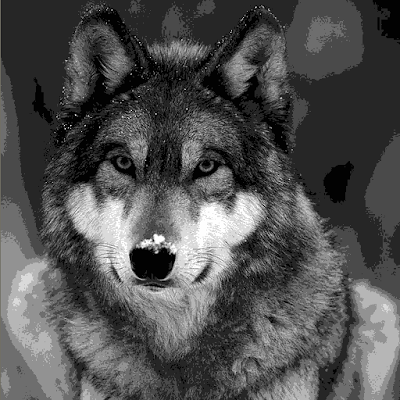## Friday, June 25, 2010

Steganography is method for hidding secret message in covert message. In this case I mean hidding secret image into covert image. So, sometimes What You See Is NOT What You Get :) How can we hide secret 3-bit image into other 24-bit image ?

Encoding procedure

1.
secret 3-bit image means that we can hide 2^3 = 8 color palette image.
So at first we need to map these 8 colors to 3-bit pattern. Lets use such mappings:
`--------------------------  RGB       | Bit pattern--------------------------2,2,2       | 0,0,038,38,38    | 0,0,174,74,74    | 0,1,0110,110,110 | 1,0,0146,146,146 | 0,1,1182,182,182 | 1,1,0218,218,218 | 1,0,1254,254,254 | 1,1,1--------------------------`

2.
Now as we have color table, we just need to get 3-bit image required pixel and encode it's 3-bit pattern into covert RGB image. One way of doing this is to define secret bit meaning as follows: 0 means covert RBG byte is even, 1 means - odd.
So according to this definition we adjust covert image RGB bytes to be even or odd - depending to required 3-bit secret pattern. For example-
if 3-bit pattern is (1,0,1) and covert RGB pixel is (140,39,16) - then it will be converted to (141,40,17).

Decoding procedure
Secret image extraction from covert image procedure is a reversal of encoding:
1. Check covert RGB bytes are even or odd.
2. From that extract 3-bit pattern.
3. Map this 3-bit pattern to 8 color palette.

Properties of this image hidding method
1. Can be used in image formats which doesn't support alpha (transparency) channel.
2. Original image looses only 3/24 = 12.5 percents of quality, which means that it is hard or impossible to spot by eyes that something is wrong with covert image.
3. Regardless of good image quality after conversion, image histogram can show some signs of payload image. Because in some cases histogram is modulated after conversion.

Now code examples. Encoding part is left as exercise to the reader :) But here it is payload image extraction code (as always in GLSL shader language):
a) Vertex Shader (we need it for correct sampling of texels)

`varying vec2 texCoord;void main(void){   gl_Position = vec4(gl_Vertex.xy, 0.0, 1.0 );   texCoord = 0.5 * gl_Position.xy + vec2(0.5);     }`

`#version 120uniform sampler2D Texture0;varying vec2 texCoord;int binaryToDecimal(in int d1, in int d2, in int d3) {   return 4*d1+2*d2+d3;}void fillColors(inout float colors) {   colors = 2./255.;   colors = 38./255.;   colors = 74./255.;   colors = 146./255.;   colors = 110./255.;   colors = 218./255.;   colors = 182./255.;   colors = 254./255.;}int Odd(in float num) {   return int(mod(num,2.)!=0.);}void main(){      float colTable;   fillColors(colTable);   vec4 col = texture2D(Texture0, texCoord);   int d1 = Odd(col.r*255.);   int d2 = Odd(col.g*255.);   int d3 = Odd(col.b*255.);   float level = colTable[binaryToDecimal(d1,d2,d3)];   gl_FragColor = vec4(level,level,level,1.0);}`

NOTE: When using this GLSL shader for image below - make sure that covert texture is rendered to screen aligned quad of EXACTLY 512x512 pixel dimensions. Otherwise you will not get hidden image, but instead you will get just noise because of incorrect pixel/texel samplings !!!

Finally,- results. This is covert image:and this is payload image extracted from above image (after extraction GLSL shader applied):Have fun in making/analyzing covert images !